# Liquid Liquid Separation

Two immiscible liquids can be separated in a three phase separator, using the difference between densities of the two phases. The separation of liquids in this ways is governed by following equation,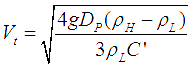where,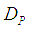= Liquid droplet diameter= Heavy liquid phase density= Light liquid phase density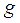= Gravitational acceleration

For a liquid droplet in another phase Reynolds number is expressed as,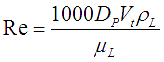is the diameter of a particle suspended in a continuous liquid phase.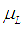= Viscosity of the continuous phase= Density of the continuous phase.

For low Reynolds numbers (less than 2), the drag coefficient (C') has a linear relationship with Reynolds number (Re). Terminal velocity equation can then be reduced to the Stokes law,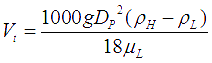The difference between two liquid densities is often low and viscosities are high, resulting in low terminal velocities. Hence, for liquid-liquid separators, the residence time required for separation is much higher than often required for gas liquid separators. Thus for high degrees of separation, liquid-liquid separators or 3 phase separators (which also involve liquid-liquid separation) require a big size.

Liquid-liquid or 3-phase separators can be either vertical or horizontal. Often horizontal separators are preferred in practical applications, as they offer a larger interphase area between two liquid phases to be separated.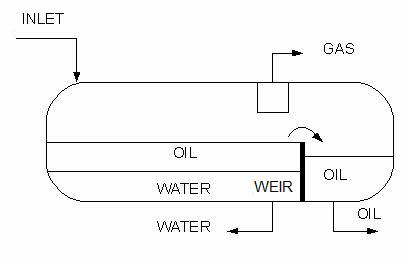Horizontal three phase separator with a weir for oil and water separation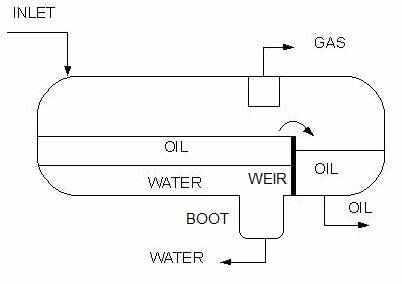Horizontal three phase separator with a boot for enhanced separation of oil from water